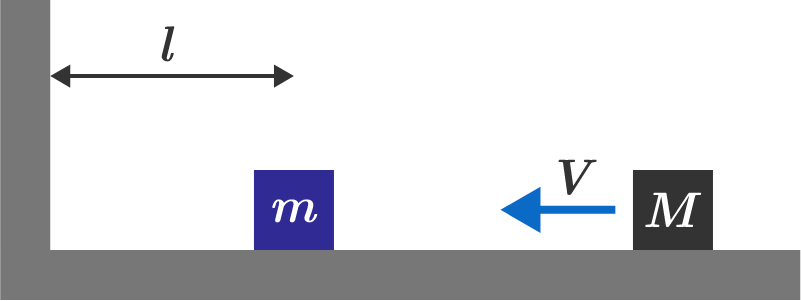# Multiple collisions

A block of mass $m$ sits on a horizontal, frictionless surface some distance $\ell$ from a wall. Another block of mass $M$ collides from the right with velocity $V.$ Depending on that ratio $x =\frac Mm,$ the blocks will collide a total of $n$ times.

Find $\displaystyle \lim_{x\rightarrow \infty} \frac{n}{\sqrt{x}}.$Assume that all collisions are elastic and that the motion is in 1-dimension.

×